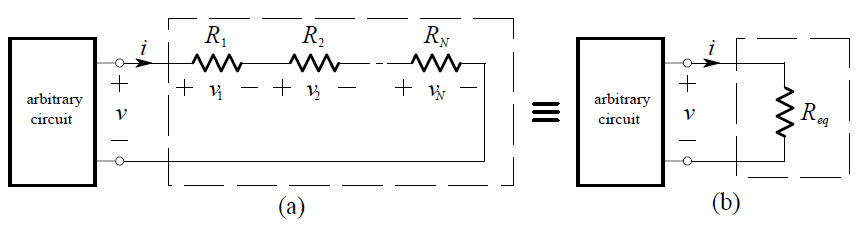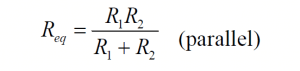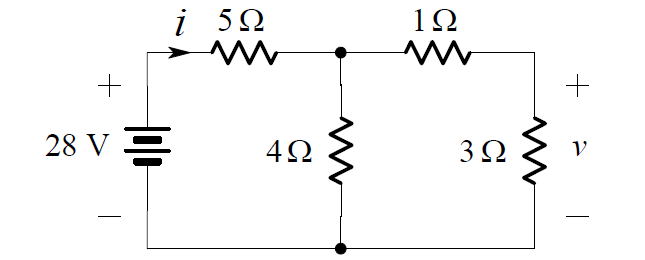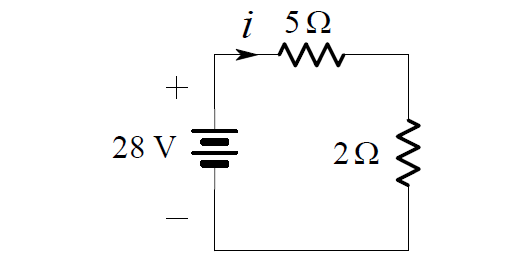# Series and Parallel Resistors

Combining Resistors

Relatively complicated resistor combinations can be replaced by a single equivalent resistor whenever we are not specifically interested in the current, voltage or power associated with any of the individual resistors.

Series Resistors

Consider the series combination of N resistors shown in (a) below:We apply KVL:

v = v1 + v2 + … vN

and Ohm’s Law:

v R1i R2i + … RN i

= (R1 + R2 + … RN )i

and  then  compare  this  result  with  the  simple  equation  applying  to  the equivalent circuit shown in above Figure

v Req . i

Thus, the value of the equivalent resistance for N series resistances is:

Req = R1 + R2 + …+ RN

Parallel ResistorsWe apply KCL:

i = i1 + i2 + … iN

and Ohm’s Law:

i G1v G2v + …GN v

= (G1  + G2+ …GN )v

whereas the equivalent circuit shown in above Figure

i Geq v

and thus the value of the equivalent conductance for N parallel conductances is:

Geq = G1 + G2 + … + GN             (parallel)

In terms of resistance instead of conductanceThe special case of only two parallel resistors is needed often:

Combining two resistors in parallelNote that since Geq  G1 + G2 then we may deduce that:

Geq  > G1  and  Geq  > G2

Hence:or:

Req  < R1   and   Req  < R2

Thus the equivalent resistance of two resistors in parallel is less than the value of either of the two resistors.

The special case of N resistors of equal value R in parallel is:Example

We want to find the current i in the circuit below:In order to find i, we can replace series and parallel connections of resistors by their equivalent resistances. We begin by noting that the 1 W are in series. Combining them we obtain: are in series. We begin by noting that the 1 ohm are 3 ohm are in series. Combining them we obtain:Note that it is not possible to display the original voltage v in this figure. Since the two 4 ohms resistors are connected in parallel, we can further simplify the circuit as shown below:Here, the 5 ohms and 2 ohms resistors are in series, so we may combine them into one 7 ohms resistor.

Then, from Ohm’s Law, we have:

i = 28/7 = 4A

Don't Miss Our Updates
Be the first to get exclusive content straight to your email.
We promise not to spam you. You can unsubscribe at any time.